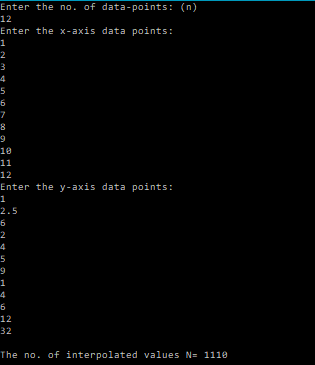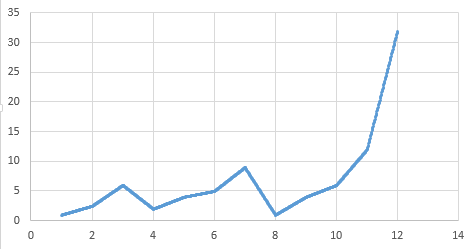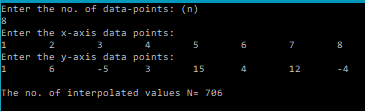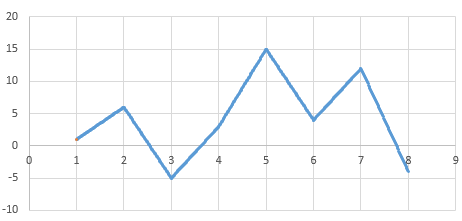# Linear Spline – Piecewise Interpolation [C PROGRAM]

Lagrange or Newton polynomial interpolations are useful interpolation techniques to have in your sleeves, but they don’t always give the best or desired result. As the degree of the polynomial increases, so do the wiggles.

Therefore, it is often advantageous to use piecewise interpolation, also known as spline interpolation.

A spline is simply a curve that connects two or more specific points.

Originally, spline was a term for elastic rulers that were bent to pass through a number of predefined points (“knots”). These were used to make technical drawings for shipbuilding and construction by hand.

In this post I will be sharing with you a C program that performs linear spline interpolation.
The user is asked to enter a set of x and y-axis data-points, and then each of these is joined by a straight line.
So the code would involve finding the equation of line connecting the two points.

You might have noticed this kind of interpolation in chart plotting softwares like Origin, Excel, Gnuplot where when you plot the data-set using the line chart, then the points are joined together by line segments as shown below.

So basically the code would need to generate a set of line segments for 2 adjacent points given by,$(x_i,y_i)$ and$(x_{i+1},y_{i+1})$.
The equation can be found by using the following formula:$y=\frac{y_{i+1}-y_i}{x_{i+1}-x_i}(x-x_i)+y_i$

So, here’s what we’re gonna do in the program:
1. We’re gonna ask the user to enter the no. of data points.
2. Then, we’re gonna let the user enter these data-points by running a simple for loop.
3. Next, we will calculate the equation of line between 2 adjacent points, and use it to interpolate the values.
4. We will store the interpolated values at a desired interval of say, 0.01, in arrays.
5. Then we will store them in a file and scatter plot them using a suitable software.

### CODE:

/*********************************
**********LINEAR SPLINE***********
*********************************/
#include<stdio.h>
/*******
Function to perform piecewise linear spline interpolation
Parameters:
n: no. of data-points
x[n]: x-axis points
y[n]: y-axis points
N: size of the array storing the interpolated x and y points
X[n]: array that stores the interpolated x-axis points
Y[n]: array that stores the interpolated y-axis points
********/
void lSpline(int n, double x[n], double y[n], int N, double h, double X[N], double Y[N]){
int i;
int j=0;
for(i=0;i<n-1;i++){
//
double yn,xn;
for(xn=x[i];xn<x[i+1];xn=xn+h){
yn=(y[i+1]-y[i])*(xn-x[i])/(double)(x[i+1]-x[i])+y[i];
//yn=(xn-x[i+1])/(x[i]-x[i+1])+(xn-x[i])/(x[i+1]-x[i]);
Y[j]=yn;
X[j]=xn;
j++;
}
}
}
main(){
int n;
int N=0; //N is the no. of interpolated values
int i;
double h=0.01; //Space interval at which interpolated values are calculated
printf("Enter the no. of data-points: (n)\n");
scanf("%d",&n);
double x[n];
double y[n];
printf("Enter the x-axis data points:\n");
for(i=0;i<n;i++){
scanf("%lf",&x[i]);
}
printf("Enter the y-axis data points:\n");
for(i=0;i<n;i++){
scanf("%lf",&y[i]);
}
//The following procedure calculates N
for(i=0;i<n-1;i++){
N=N+(x[i+1]-x[i])/h;
}
//A little adjustment to get the correct value of N
N=N+2+(n-4);
printf("\nThe no. of interpolated values N= %d\n",N);
double Y[N];
double X[N];
//Perform piece-wise linear interpolation
lSpline(n,x,y,N,h,X,Y);
//Store the interpolated values in a File
FILE *fp = "NULL";
fp=fopen("linSpline1.txt","w");
for(i=0;i<N;i++){
fprintf(fp,"%lf\t%lf\n",X[i],Y[i]);
}
}



### OUTPUT:### Android App:

I’ve created a few data interpolation android apps, that you can check out.